Checkout JEE MAINS 2022 Question Paper Analysis : Checkout JEE MAINS 2022 Question Paper Analysis :

# Oxidation Number

Oxidation number in simple terms can be described as the number that is allocated to elements in a chemical combination. The oxidation number is basically the count of electrons that atoms in a molecule can share, lose or gain while forming chemical bonds with other atoms of a different element.

Oxidation number is also referred to as oxidation state. However, sometimes these terms can have a different meaning depending on whether we are considering the electronegativity of the atoms or not. The oxidation number term is used frequently in coordination chemistry.

In general, oxidation state or number helps us describe the transfer of electrons. However, students have to note that it is different from a formal charge which determines the arrangement of atoms. The oxidation number/state is also used to determine the changes that occur in redox reactions. Meanwhile, it is quite similar to valence electrons.

## What is Oxidation Number?

Oxidation number of an atom is defined as the charge that an atom appears to have on forming ionic bonds with other heteroatoms. An atom having higher electronegativity (even if it forms a covalent bond) is given a negative oxidation state.

The definition, assigns oxidation state to an atom on conditions, that the atom –

i) Bonds with heteroatoms.

ii) Always form ionic bonding by either gaining or losing electrons, irrespective of the actual nature of bonding.

Since an atom can have multiple valence electrons and form multiple bonds, all of them will be, assumed to be ionic and assigned oxidation state equal to the number of electrons involved in the bonding. So, oxidation number or state is, a hypothetical case of assumption of atoms forming an ionic bond.

In the complex cation, tetroxoplatinum (PtO4)2+, Platinum possess an oxidation state of 10. Ten is the maximum oxidation state exhibited by any atom. It appears to have lost ten electrons to form the ion. But, the ionization energy required for removing an electron from charges positively species increases heavily.

Larger the charge, it is difficult to remove an electron and so, higher the ionization energy. So, the removal of ten electrons is highly hypothetical. Similarly, the addition of electron also becomes difficult with increasing negative charge.

Oxidation states, larger than three, whether positive or negative are practically impossible. In spite of the assumption, it helps in understanding the changes accompanying the atom undergoing a chemical change.

## How To Find Oxidation Number Of An Atom?

Oxidation number or state of an atom/ion is the number of electrons an atom/ion that the molecule has either gained or lost compared to the neutral atom. Electropositive metal atoms, of group I, 2 and 3 lose a specific number of electrons and have always constant positive oxidation numbers.

In molecules, more electronegative atom gain electrons from a less electronegative atom and have negative oxidation states. The numerical value of the oxidation state is equal to the number of electrons lost or gained.

Oxidation number or oxidation state of an atom or ion in a molecule/ion is assigned by:

i) Summing up the constant oxidation state of other atoms/molecules/ions that are bonded to it and

ii) Equating, the total oxidation state of a molecule or ion to the total charge of the molecule or ion.

### Video on Paradox of Fractional Oxidation Number## Atoms/ Molecules and Ions that have Constant Oxidation State (Number)

a) The net charge on neutral atoms or molecules is zero. So the overall oxidation state of them is zero.

For example oxidation state of elemental atoms such as sodium, magnesium, iron is zero. Similarly, the net oxidation state of neutral molecules such as oxygen, chlorine, water, ammonia, methane, potassium permanganate is zero.

The oxidation state of atoms in homo-polar molecules is zero. The oxidation number of an atom in an oxygen molecule is zero.

b) The oxidation state of charged ions is equal to the net charge of the ion. So,

1. Oxidation number of all alkali metal ions is always = +1
2. Oxidation number of all alkaline earth metal ions is always = +2
3. Oxidation number of all boron family metal ions is always = +3
4. Oxidation number of hydrogen in proton (H+) is +1, and in hydride is -1.
5. Oxidation number of oxygen in oxide ion(O2-) is -2, and in peroxide ion(O-O2-) is -1.

## Calculation of Oxidation Number of an Atom in a Molecule/Ion

Oxidation number of potassium permanganate (KMnO4) = Sum of oxidation number of (K + Mn + 4O) = 0

Oxidation number of permanganate ion (MnO4) = Sum of oxidation number of ( Mn + 4O)= -1

## Calculation of Oxidation Number of atoms Occurring Only Once in a Molecule

Examples 1: Oxidation state of chlorine in KCl

KCl is neutral and so, net charge=0

Oxidation state of KCl = Oxidation state of potassium + oxidation state of chlorine = 0.

Oxidation state of potassium = +1

Oxidation states → +1 + x = 0: x = -1

Atoms in the species → K Cl

Oxidation state of chlorine in KCl = -1

Example 2: Oxidation number of Manganese in permanganate ion MnO4

Charge on the permanganate ion is -1

Oxidation state of permanganate ion =Oxidation state of manganese + 4 oxidation state of oxygen = -1.

Oxidation state of oxygen = -2

Oxidation states → x + (4*-2) = -1: x = +7

Atoms in the species → Mn 4O

Oxidation state of manganese = +7

Example 3: Oxidation number of a metal ion in a complex.

i) Ni(CO) 4.

The total charge of the complex is zero. CO is a neutral molecule.

Oxidation states → x + (4*0) = 0: x = 0

Atoms in the species → Ni 4 CO

Nickel is also in zero oxidation state.

ii) [CoCl2(NH3)4]Cl.

The complex can be written in the ionic forms as [CoCl2(NH3)4]+Cl.

Metal is in a cationic complex with a unitary positive charge. Ammonia is a neutral ligand and chlorine has a unit negative charge.

Oxidation number of [CoCl2(NH3)4]+ = Oxidation number of (Co + 2Cl + 4×0) = +1.

Oxidation states → x + (2*-1) + 4*0 = +1: x = +3

Atoms in the species → Co 2Cl 4 NH3

Oxidation number of cobalt in the complex = +3

## Calculation of Oxidation Number of Atoms Occurring More Than Once in a Molecule and Having Identical Bonding

Atom occurring ore than in a molecule may be, bonded in an identical way or not. If they are identically bonded, then there is no difference between them, and all the atoms will have the same oxidation numbers. The oxidation state of such an atom in a molecule can be, calculated by the normal method.

The average oxidation number will be the same as calculated individually and a whole number.

Example 1: The number of atoms of chlorine is two in the molecules Cl2O, Cl2O5 and Cl2O7. But, the environment of both atoms of chlorine is the same as shown by their structures. The oxidation number of the atoms calculated either individually or from the whole molecule is the same.

i) Cl2O: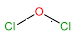Cl2O is neutral and so, net charge=0.

Net oxidation state of Cl2O = 2 x Oxidation state of chlorine + 1x Oxidation state of oxygen = 0.

Oxidation state of oxygen = -2.

Oxidation states → 2 x + (-2) = 0: x = +1

Atoms in the species → 2Cl O

Oxidation state of chlorine in Cl2O=

$$\begin{array}{l}\frac{2}{2}\end{array}$$
= +1

ii) Cl2O5: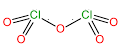Cl2O5 is neutral and so, net charge=0

Oxidation state of Cl2O5 = 2 x Oxidation state of chlorine + 5 x oxidation state of oxygen = 0.

Oxidation state of oxygen = -2.

Oxidation states → 2x + (5*-2) = 0: x = +5

Atoms in the species → 2Cl 5O

Oxidation state of chlorine in Cl2O5 =

$$\begin{array}{l}\frac{10}{2}\end{array}$$
= +5

iii) Cl2O7: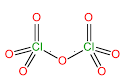Cl2O7 is neutral and so, net charge=0

Oxidation state of Cl2O7 = 2 x Oxidation state of chlorine + 7 x oxidation state of oxygen = 0.

Oxidation state of oxygen = -2.

Oxidation states → 2x + (7*-2) = 0: x = +7

Atoms in the species → 2Cl 7O

Oxidation state of chlorine in Cl2O =

$$\begin{array}{l}\frac{14}{2}\end{array}$$
= +7

Note: Except the atoms/molecules/ions mentioned, as having a constant oxidation state, oxidation state of other atoms/molecule and ions will vary depending on the molecule they are present.

In the given examples, the oxidation state of chlorine is not constant, but variable (+1, +5 and +7)

Example 2: Oxidation state of chromium in dichromate anion.

Dichromate ion is Cr2O72-.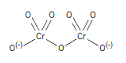Charge on the ion is -2.

Oxidation state of dichromate ion = 2 x Oxidation state of chromium + 7 x oxidation state of oxygen = -2.

Oxidation state of oxygen = -2.

Oxidation states → 2x + (7*-2) = -2: x = +6

Atoms in the species → 2Cr 7O

Oxidation state of chromium= 12 / 2 = 6

## Calculation of Oxidation Number of Atoms Occurring More than Once in a Molecule and Having a Difference In Bonding

Atoms having different bond structure will have different oxidation state. Hence, their oxidation state has to be individually determined from their molecular structure. Average oxidation state can be calculated by assuming them to be equal. In such a case, the average oxidation could be fractional rather than a whole integer.

Example 1: Cl2O4

i) The average oxidation state of chlorine

Cl2O4 is neutral and so, net charge = 0

Oxidation state of Cl2O4 = 2 x Oxidation state of chlorine + 4 x oxidation state of oxygen = 0. ⸪

Oxidation state of oxygen = -2.

Oxidation states → 2x + (4*-2) = 0: x = +4

Atoms in the species → 2Cl 4O

Oxidation state of chlorine in Cl2O5 =

$$\begin{array}{l}\frac{8}{2}\end{array}$$
= +4

ii) Structure of Cl2O4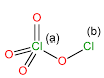Individual oxidation state of oxygen ‘a’ is +7

Individual oxidation state of oxygen ‘b’ is +1

None of the oxygen has a +4 oxidation state.i) Average oxidation number of carbon

Five carbon atoms share the five electrons from five hydrogen atoms and additional electron of the negative charge by resonance. So, six electrons are shared by five-carbon.

Average oxidation state of each carbon =

$$\begin{array}{l}\frac{6}{5}\end{array}$$
= fraction

ii) Without resonance, four carbon has -1 oxidation state and one carbon has -2 oxidation state.

## Oxidation Number of Atoms in a Diatomic Molecule

A diatomic molecule can be either homo or heteronuclear.

i) Homonuclear diatomic molecule:

Oxidation number concept is applicable only to heteroatoms forming a molecule. Hence, in a homonuclear diatomic molecule, the oxidation number of the atoms is zero. The oxidation number of hydrogen or oxygen, nitrogen, chlorine in respective molecules is zero.

ii) Heteronuclear diatomic molecule:

In hetero diatomic molecules, all bonds formed between the atoms are, considered as ionic.

More electronegative atoms are assumed to take away the bonding electrons from the less electronegative atom. So, the electronegative atom will have a negative oxidation state and the magnitude is equal to the number of electrons taken by it.

The less electronegative atom is supposed to have lost its electron to the more electronegative atom. So, the less electronegative atom will have a positive oxidation state equal to the number of electrons lost by it.

Example 1: HCl

Chlorine is highly electronegative than hydrogen. So, chlorine is, assumed to take away the electron from hydrogen. Chlorine, which receives one electron, has an oxidation number of -1, while hydrogen losing one electron has an oxidation state of +1.

Example 2: H2O

Oxygen is more electronegative than hydrogen. So, the oxygen atom receives one electron each from the two-hydrogen atom and will have an oxidation number of -2. Both hydrogens losing one electron each will have an oxidation number of +1 each.

## Fractional Oxidation States

Oxidation state is the number of electrons assumed to have either lost or taken by heteroatoms during their bonding. Since the numbers of electrons are whole numbers, the oxidation number of individual atoms also has to be a whole integer.

But, there are molecules that contain an atom, more than once and each bonded differently. Such atoms shall have different oxidation state at different positions and hence has to be, calculated individually, taking into consideration of the atoms it bonds.

Calculation of the oxidation state of the atom using the normal method assumes all the same atom as equal and will give only an average of the different oxidation states of the same atom in the molecule. This, average oxidation state, is mostly a fraction, instead of the whole number.

So, the fractional oxidation state is always an average oxidation number of the same atoms in a molecule and does not reflect the true state of the oxidation state of atoms.

Example 1: Superoxide -KO2

Potassium ion has an oxidation number of +1. Potassium superoxide molecule being neutral, the oxidation state of two oxygen atoms together is -1.

So, average oxidation number of oxygen in super oxide is

$$\begin{array}{l}-\frac{1}{2}\end{array}$$
.

Structure of superoxide ion is;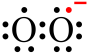As per the structure, one oxygen atom has zero oxidation state. The second oxygen atom is negatively charged and has -1 oxidation state. So, the true oxidation state of oxygen atoms is not minus half each but 0 and -1.

Example 2: Fe3O4

Considering the oxidation state of oxygen as -2, the average oxidation state of iron atoms will be

$$\begin{array}{l}+\frac{8}{3}\end{array}$$
.

But the molecule is a mixture of two compounds of FeO and Fe2O3.

In FeO and Fe2O3 iron is in +2, and +3, oxidation states. So, in Fe3O4, one iron has +2 and to iron has +3 oxidation states.

Average oxidation state is =

$$\begin{array}{l}+\frac{2+3+3}{3} = +\frac{8}{3}\end{array}$$

Example 3: Tetra-thionate ion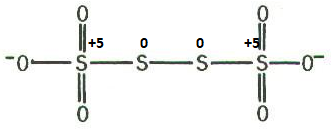Tetrathionate ion has four sulphur atoms bonded to oxygen as in the structure. Out of the four sulphur atoms, the two-terminal sulphur atoms are, connected to three oxygen heteroatoms and one homo sulphur atom. Each terminal sulphur atom forms five bonds with oxygen heteroatoms and so the oxidation state will be +5. The bridging sulphur atoms being homo-nuclear have zero oxidation state.

Total oxidation of the entire four Sulphur atoms is ten.

So, average oxidation state of Sulphur =

$$\begin{array}{l}\frac{10}{4}\end{array}$$
= 2.5

## Oxidation and Reduction, Redox Reactions

Atoms and molecules react to form products. The reactions are, classified into many types based on the nature of change on the reactants to form products. Whatever may be the reaction types, reactant and product atoms/ions in the reaction may either have the same or a different number of valence electrons.

Reactions, where the number of valence electrons in the reactant atom/ion, is different from the product side are, called as reduction-oxidation or simply redox reactions. Atom/ion might have either lost or gained electrons during the reaction. Accordingly, atom/ion is, said to be either oxidized or reduced.

Atoms/ions in the reactions are represented by their atomic symbol with a superscript. The superscript represents the difference in the number of electrons of the atom /ion compared to the neutral atom. The superscript also has a positive sign if the electron is lost and a negative sign if the electron is gained compared to the neutral atom.

The superscript along with the sign is, called ‘oxidation state’ of the atom. The atom may have different oxidation states depending upon the number of electrons either gained or lost. Neutral atoms have zero oxidation state.

 Reaction Reaction type The oxidation state of the atom in the reactant side The oxidation state of the atom in the product side M0 → M+ + e– Oxidation reaction 0 +1 M+ + e– → M0 Reduction +1 0 M2+ → M3+ + e– Oxidation reaction +2 +3 M3+ + e– → M2+ Reduction +3 +2

In redox reactions:

In redox reactions, atoms or ions either loss or gain electrons and have different oxidation states, before and after the reaction.

• Oxidation number can be positive or zero or negative
• Oxidation number has to be an integer as the number of electrons can only be an integer.
• Oxidation number cannot be fractional
• The oxidation number is the same as the oxidation state.

## Frequently Asked Questions on Oxidation Number

### What is the oxidation number of alkali and alkaline earth metals?

The oxidation number of alkali and alkaline earth metals is +1 and +2 respectively.

### What is the oxidation number of oxide ions?

The oxidation number of oxide ions is -2.

### What is the oxidation number of hydrogen in HCl?

The oxidation number of hydrogen in HCl is +1.
Test Your Knowledge On Oxidation Number!